## Excel TRANSPOSE Function

Excel TRANSPOSE formula allows you to rotate (swap) values from rows to columns and vice versa in Excel. Its goal, as a component of the Excel lookup and reference functions, is to arrange data in the appropriate manner. To run the formula, pick the precise size of the transposed range and hit the CSE key (“Control+Shift+Enter“).

## Description

The TRANSPOSE function in Microsoft Excel returns a transposed range of cells. For instance, if a vertical range is specified as a parameter, a horizontal range of cells is returned. Alternatively, if a horizontal range of cells is specified as a parameter, a vertical range of cells is returned.

Excel TRANSPOSE formula is an Excel built-in function that is classified as a Lookup/Reference Function. It may be used in Excel as a worksheet function (WS). The TRANSPOSE function is a worksheet function that may be used as part of a formula in a worksheet cell.

## Syntax

The Excel TRANSPOSE Function in Microsoft Excel has the following syntax:

`=TRANSLATE (range)`

Arguments or Parameters:

range – The range of cells to transpose.

## Returns

TRANSPOSE returns a range of cells that have been transposed.

Note:

The TRANSPOSE function requires an array as the range value. Enter a value and then press Ctrl+Shift+Enter to create an array. This will enclose the formula in brackets, indicating that it is an array.

## Applicable To

Office 365

Notes on use:

• The TRANSPOSE function transforms a vertical range of cells to a horizontal range of cells, or vice versa. TRANSPOSE, in other terms, “flips” the orientation of a specified range or array:
• TRANSPOSE translates a vertical range to a horizontal range when provided one.
• TRANSPOSE transforms a horizontal range to a vertical range when provided one.
• When an array is transposed, the first row becomes the new array’s first column, the second row becomes the new array’s second column, the third row becomes the new array’s third column, and so on.
• TRANSPOSE is a function that works with both ranges and arrays. Ranges that have been transposed are dynamic. If the data in the source range changes, TRANSPOSE updates the data in the target range instantly.

## Excel TRANSPOSE Function

The TRANSPOSE Excel function, as the name implies, may be used to transpose data in Excel.

The syntax is as follows:

`=TRANSPOSE(array)`

The array denotes a range of cells to be transposed in this case.

Take the following basic steps:

Step1: Choose the range of cells in which you wish the output data to be transposed.

Step2: TRANSPOSE is an array formula; hence, the precise amount of cells must be specified.

Step3: If the range of your table is 6×2, i.e., 6 rows and 2 columns, you must pick a 2×6 range for the transposed data, i.e., 2rows and 6 columns.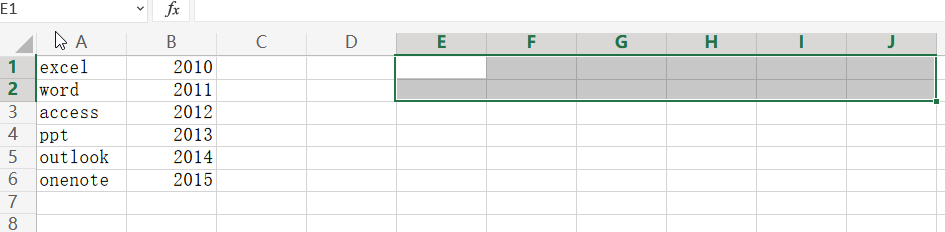Step4: Insert the formula =TRANSPOSE (A1:F5)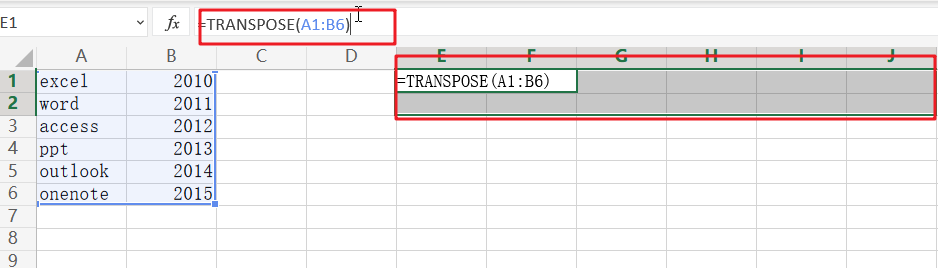Step5: Avoid pressing Enter! Ctrl+Shift+Enter is required to perform array formula.

Step6: It’s worth noting that transpose is an array formula. An array formula must be input by hitting the `Ctrl+Shift+Enter` key combination, which causes the formula bar to insert a set of braces around the formula.

Step7: This instructs Excel to print an array of data rather than a single cell.

Step8: Transposition of the data is now complete.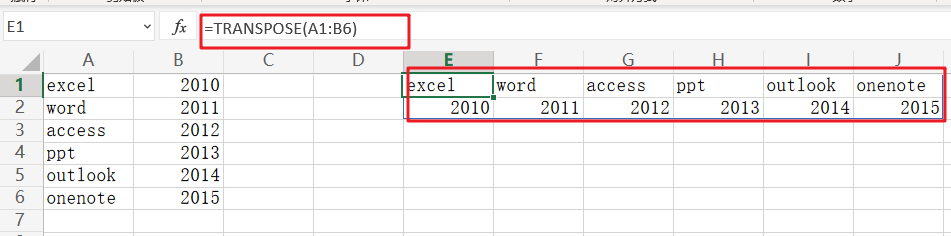## Extract or Filter Horizontal Data

You might have been through this kind of situation where you need to filter out the horizontal data from the list having few columns. I am also pretty sure about it that you might have chosen to do it manually, which is also a great choice when you have only a few values in a list, and you want to filter out the top n values.

However, if you are dealing with numerous columns in the list and want to filter out the horizontal data, then executing these jobs manually would be a stupid move since there is a 90% probability that you will become weary of it and will be unable to accomplish your assignment on time.

But don’t worry about it, since after attentively reading this post, filtering out horizontal data from a list with many values will be a piece of cake for you.

So let’s go into the article and solve this problem.

#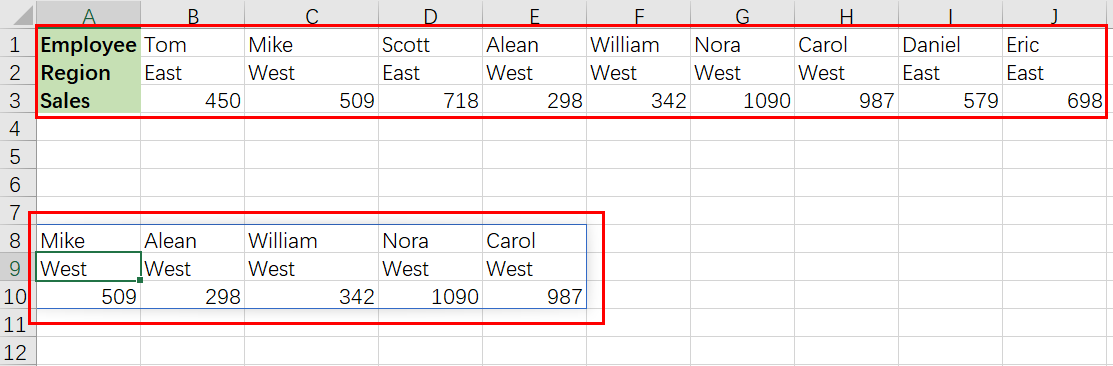## General Formula

The Following formula would help you Filter the horizontal data in MS Excel:

`=FILTER(data_range,criteria)`

## Explanation of Syntax

Before we go into the formula for getting the job done quickly, we need to understand each syntax to comprehend how each syntax helps filter out the horizontal data in MS Excel.

• `Filter`: This function contributes to narrowing down or filtering out a range of data on the user-defined criteria.
• `data_range`: In your worksheet, it represents the input ranges.
• `Comma symbol (,)`: In Excel, this comma symbol acts as a separator that helps to separate a list of values.
• `criteria`: the criteria on which you want to collect or filter the data.
• `Parenthesis` (): The core purpose of this Parenthesis symbol is to group the elements and separate them from the rest of the elements.

## Let’s See How This Formula Works

For understanding easily that how this formula works and how to use this formula, Suppose that you got a task in which three horizontal rows have multiple columns, each horizontal row have different types of data; now you want to filter out data from these horizontal rows on a certain logic or criteria.

As to filter the horizontal data on a certain logic, we would write the formula according to the given list like:

` =FILTER(data_range,region="West")`

#Here in this formula, the named ranges are `data_range`` (B1:J3)` and `region`` (B2:J2)`

Please remember that FILTER is a new dynamic array function in Excel 365. There are equivalents in other versions of Excel, but they are more Sophisticated.

The primary goal is to filter this horizontal data to extract only columns (records) with the region “West“.

The FILTER function can extract data that is arranged vertically (in rows) or horizontally (in columns). The matching data will be returned in the same orientation by the FILTER function.

There is no special setup required. The formula in A8 in the example shown is:

`=FILTER(data_range,region="West")`

The included argument for FILTER is a logical expression that works from the inside out:

`=region="West"/ look for the word "West "`

When the logical expression is evaluated, it yields an array of ten TRUE and FALSE values:

` {FALSE,TRUE,FALSE,TRUE,TRUE,TRUE,TRUE,FALSE,FALSE}`

#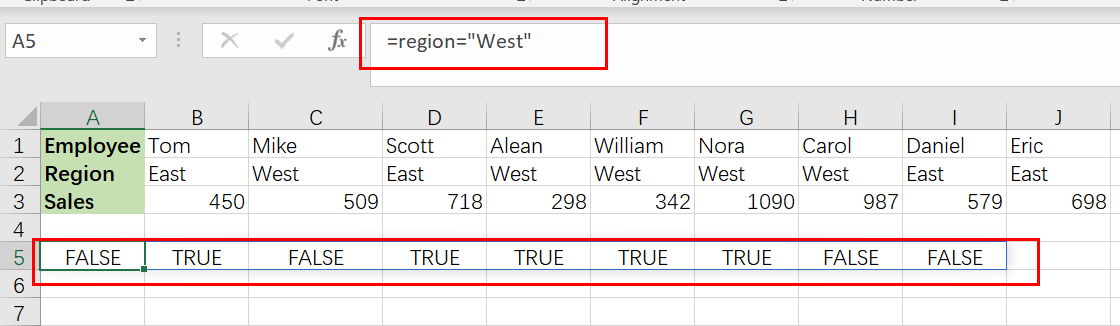It’s worth noting that the commas (,) in this array indicate columns. Rows would be indicated by semicolons (;).

The array has one value for each column of data, and each TRUE corresponds to a column with the region “ West” .

This array is passed straight to FILTER as the included parameter, and it conducts the filtering of the data:

`=FILTER(data_range,{ FALSE,TRUE,FALSE,TRUE,TRUE,TRUE,TRUE,FALSE,FALSE })`

#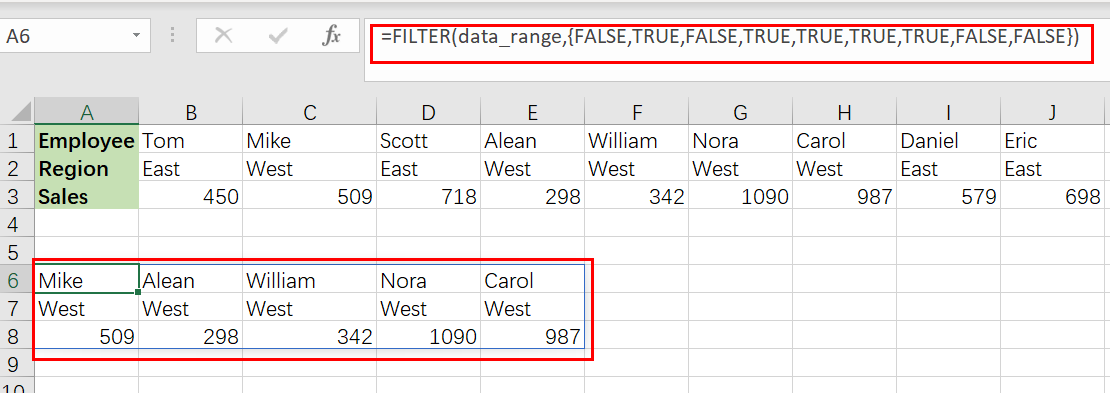Because the filter only accepts data corresponding to TRUE values, FILTER delivers the 5 columns where the region is “ West“.

This data is returned by FILTER in its original horizontal layout.

## Transpose Filter Results

If you want to transpose the filter results into a vertical  format, you can use the TRANSPOSE function around the FILTER function like follows:

`=TRANSPOSE(FILTER(data_range,region="West"))`

The result is as follows:

#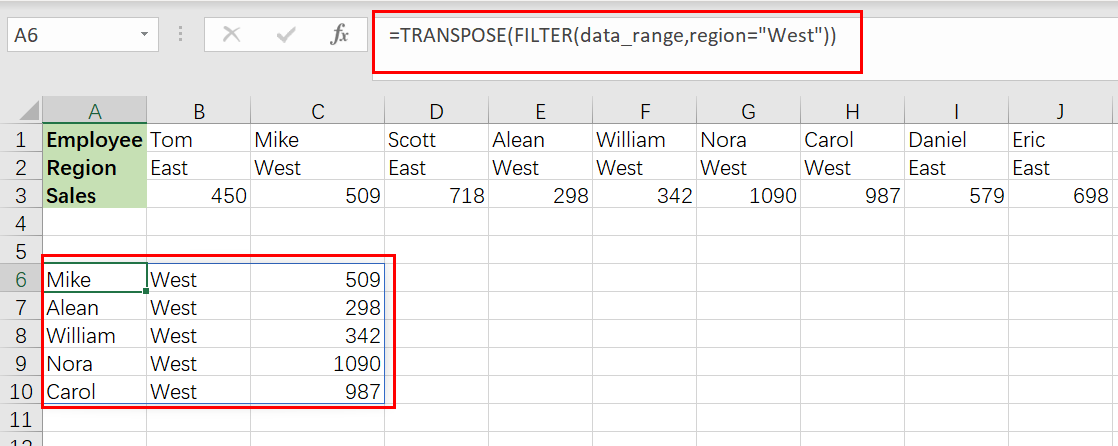## Filter Data by Another Column

The same fundamental method may be used to filter data in a variety of ways. For instance, if you want to filter out data to show only columns with sales greater than 600, just use the following formula based on the FILTER function:

`=FILTER(data_range,B3:J3<600)`

#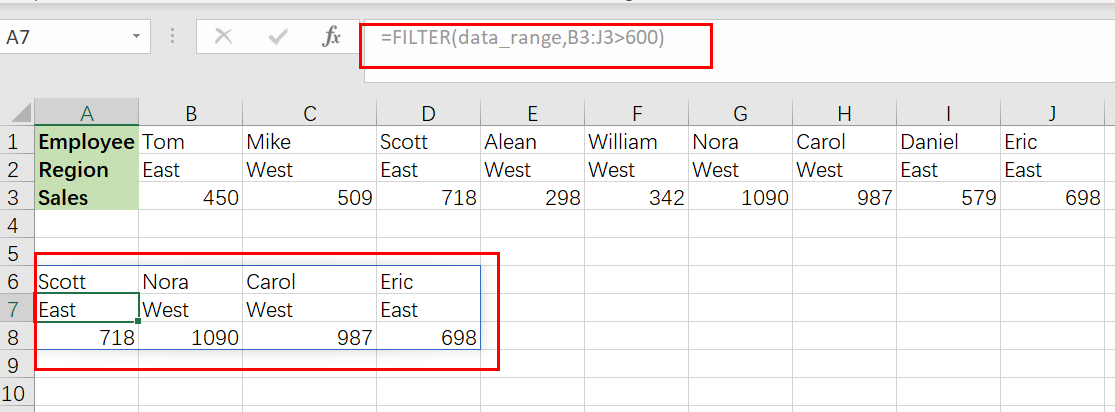### Related Functions

• Excel Filter function
The Excel  FILTER function extracts matched records from a collection of data using one or more logical checks. The include argument specifies logical tests, which might encompass a wide variety of formula conditions.==FILTER(array,include,[if empty])…
• Excel TRANSPOSE function
Excel TRANSPOSE formula allows you to rotate (swap) values from rows to columns and vice versa in Excel.The Excel TRANSPOSE Function syntax:=TRANSLATE (range) …

## Filter And Transpose Data From Horizontal To Vertical

This post will show you how to use Filter function and in combination with Transpose function to filter data from horizontal and transpose data as vertical in Microsoft Excel.

You can refer to the below general formula based on TRANSPOSE and FILTER function:

`=TRANSPOSE(FILTER(range,logic))`

You can use the FILTER with TRANSPOSE function to filter data horizontally and show the result in a vertical format. In the below example, the formula in A8 is used:

`=TRANSPOSE(FILTER(\$B\$1:\$J\$3,\$B\$1:\$J\$1=2))`

## Clarification

This task will filter the horizontal data in range `\$B\$1:\$J\$3`, and display results transposed to a vertical format. We will work with both the data range (`\$B\$1:\$J\$3`) and Class ID range (`\$B\$1:\$J\$1`) in this example.

Filters are one of the most useful functions for organizing your data. You can use them to extract specific pieces or ranges, and they’ll return only what you want! For example, the formula in A8 is:

`=TRANSPOSE(FILTER(\$B\$1:\$J\$3,\$B\$1:\$J\$1=2))`

The argument includes filter can be used to check for the existence of a file or files. This means it will only return true when the input string matches one or more pattern(s). The syntax looks like this:

`\$B\$1:\$J\$1=2`

The output is an array of 9 TRUE and FALSE values.

`{FALSE,TRUE,FALSE,FALSE,TRUE,FALSE,FALSE,TRUE, FALSE }`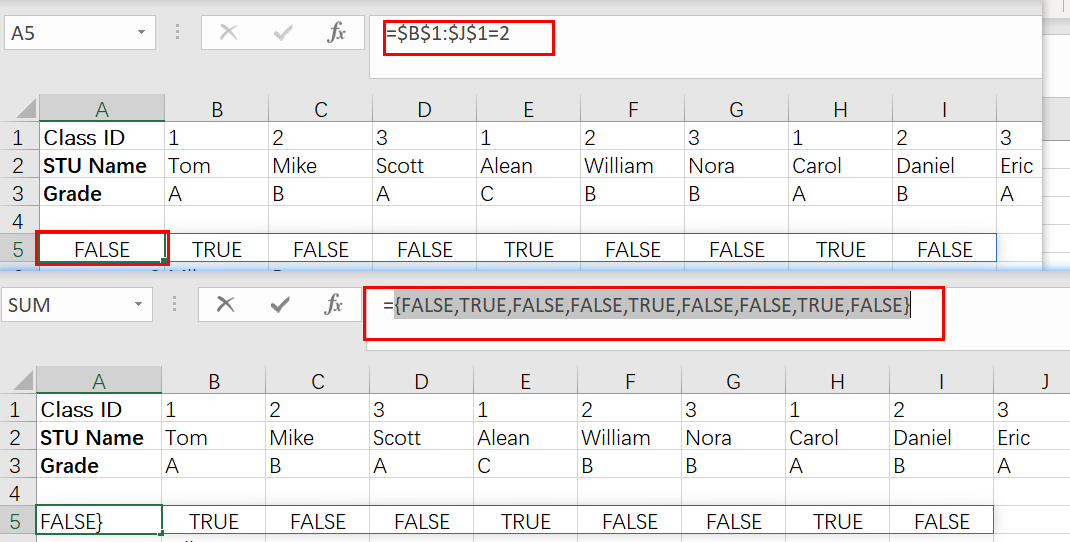The input array contains one value per record in the data, and each TRUE corresponds to a “2” Class ID column. This returning value is then used by FILTER as an argument for its filtering:

`FILTER(\$B\$1:\$L\$3,{FALSE,TRUE,FALSE,FALSE,TRUE,FALSE,FALSE,TRUE, FALSE })`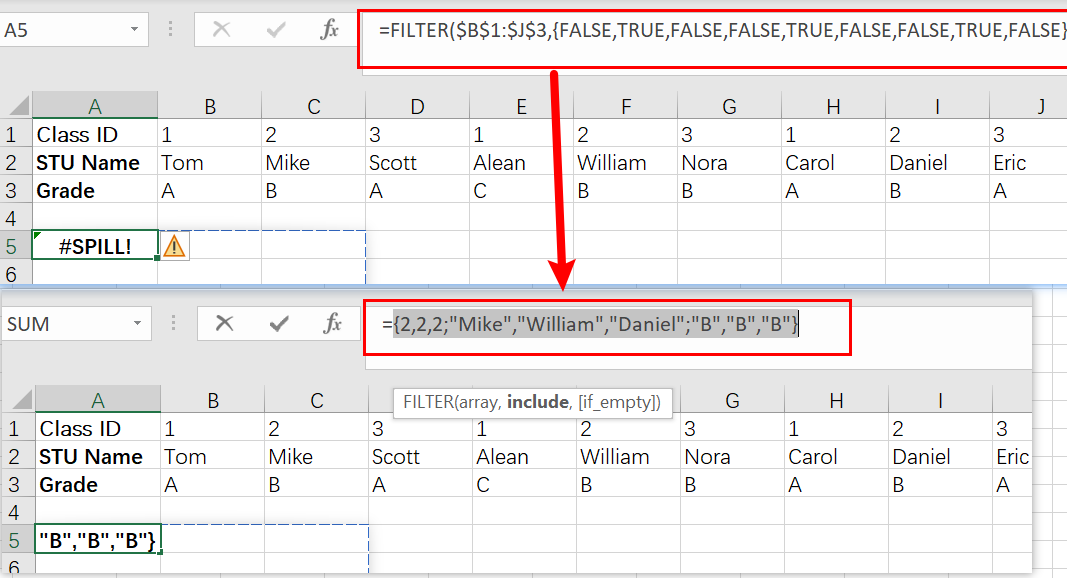By only passing through columns that correspond with TRUE, the result is a set of data for those three people in  Class 2. FILTER gives us back this original format but displays it vertically instead! The TRANSPOSE function was included so we can see they’re displayed properly.

`=TRANSPOSE(FILTER(\$B\$1:\$J\$3,\$B\$1:\$J\$1=2))`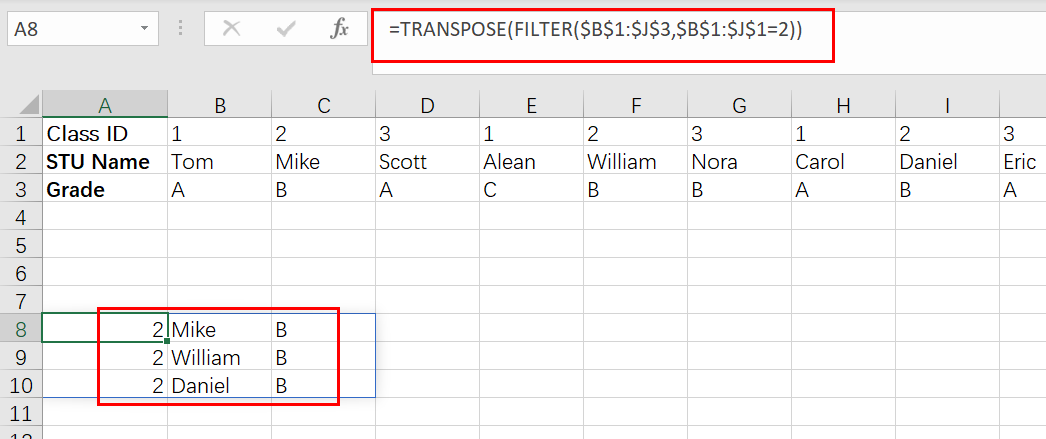By using the TRANSPOSE function, we can transform our data into a vertical array. This means that when there is a variation in any of cells B1 through J3 (or their values), all filter results will be updated automatically!

### Related Functions

• Excel Filter function
The Excel  FILTER function extracts matched records from a collection of data using one or more logical checks. The include argument specifies logical tests, which might encompass a wide variety of formula conditions.==FILTER(array,include,[if empty])…
• Excel TRANSPOSE function
Excel TRANSPOSE formula allows you to rotate (swap) values from rows to columns and vice versa in Excel.The Excel TRANSPOSE Function syntax:=TRANSLATE (range) …

## How to Count Row That Contain Specific Value in Excel

This post will guide you how to count rows that contain a specific text or number in Excel 2013/2016 or Excel office 365. How do I count the number of rows that contain a particular value while working with your current worksheet in Excel. And you can do this by a array formula based on the SUM function, the MMULT function, the TRANSPOSE function and the COLUMN function in Excel.

## General Formula:

The below general formula can help you to count rows that have specific values in Excel. Like this:

`=SUM(--(MMULT(--(criteria), TRANSPOSE(COLUMN(Range)))>0))`

Note: this formula is an array formula and you have to press “`CTRL + SHITF +Enter`” to change it from a normal formula to a array formula.

## Count Row That Contain Specific Value

Assuming that you have a data of list in range B2:C6. And you want to know the number of rows that have a particular value 60 in your given range of cells.  And you can refer to the above generic formula to create a new array formula. Like this:

`=SUM(--(MMULT(--(B1:C6=60),TRANSPOSE(COLUMN(B1:C6)))>0))`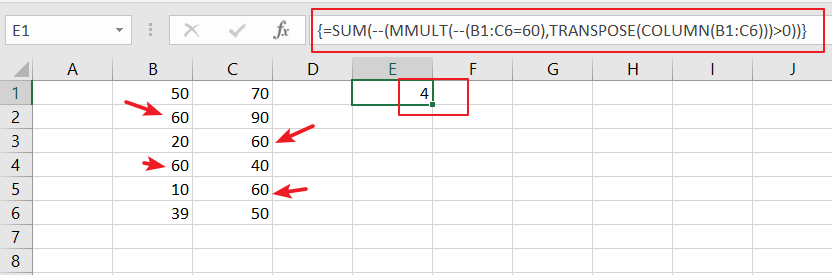Let’s See That How This Formula Works:

`=--(B2:C6=60)`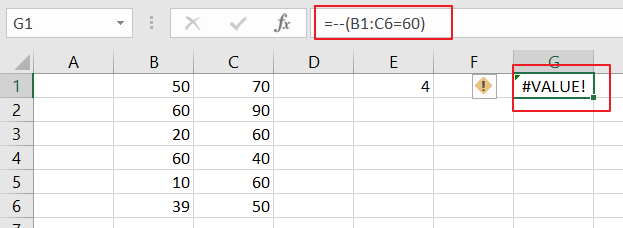This formula is a logic criteria, and it is used to generate a TRUE and FALSE array result, and the double negative operator can be used to force the TRUE and FALSE values to 1 and 0 respectively.

`{0,0;1,0;0,1;1,0;0,1;0,0}`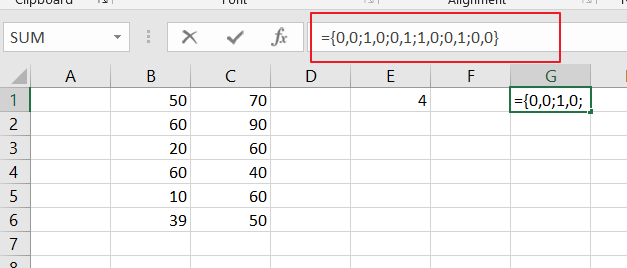The Column function can be used to get the column number in an array format. And the TRANSPOSE function is used to change the column array format to row array.

Finally, the SUM function will count all those rows that have your specific value in the given range of cells.

Related Functions

• Excel COLUMN function
The Excel COLUMN function returns the first column number of the given cell reference.The syntax of the COLUMN function is as below:=COLUMN ([reference])….
• Excel SUM function
The Excel SUM function will adds all numbers in a range of cells and returns the sum of these values. You can add individual values, cell references or ranges in excel.The syntax of the SUM function is as below:= SUM(number1,[number2],…)…
• Excel TRANSPOSE function
Excel TRANSPOSE formula allows you to rotate (swap) values from rows to columns and vice versa in Excel.The Excel TRANSPOSE Function syntax:=TRANSLATE (range) …

## How to Transpose Rows and Columns in Excel

This post will guide you how to convert row data to columns in Excel. How do I convert columns to rows with Paste feature in Excel 2013/2016.

## Convert Rows to Columns Using Paste Special

Assuming that you have a list of data in range A1:D5, and you want to change columns to rows for your data in the current worksheet in Excel. You can use the Paste Special feature to achieve the result. Just do the following steps:

#1 select the range of cells that you want to transpose.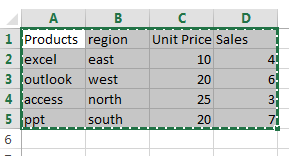#2 press Ctrl + C keys on your keyboard to copy the selected cells.

#3 select one black cell as the first cell of the destination range. And right click on it and select Paste Special from the popup menu list. And the Paste Special dialog will open.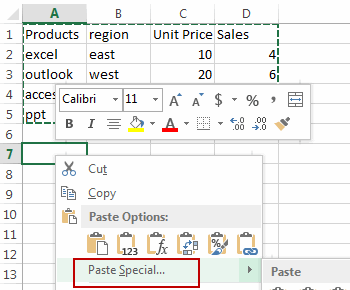#4 select Transpose checkbox in the Paste Special dialog box. And click OK button.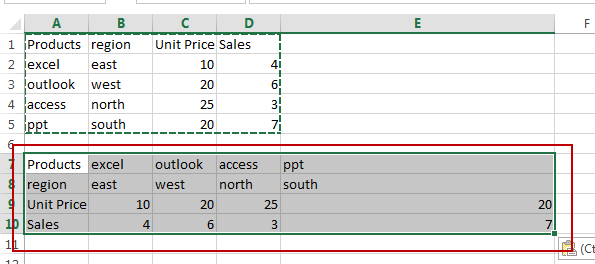## Convert Rows to Columns Using Formula

You can also use a formula based on the TRANSPOSE function to quickly convert columns to rows in Excel. Here are the steps:

#1 you need to count the number of rows and columns in your range A1:D5 and select the same number of blank cells (4 rows and 5 columns)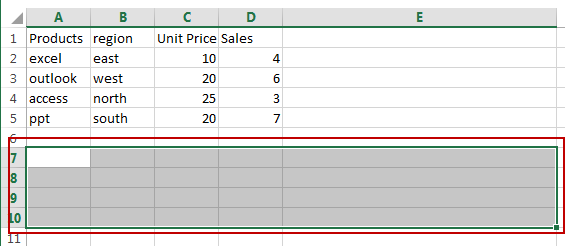#2 type the following formula in the first empty cell of your selected range of cells.

`=TRANSPOSE(A1:D5)`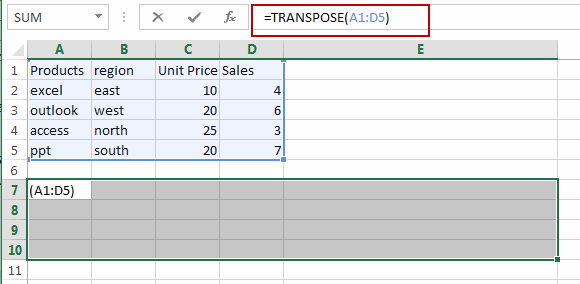#3 then press Ctrl + Shift + Enter keys on your keyboard to make it an Excel Array formula. You would notice that the columns are converted to rows for your original data A1:D5.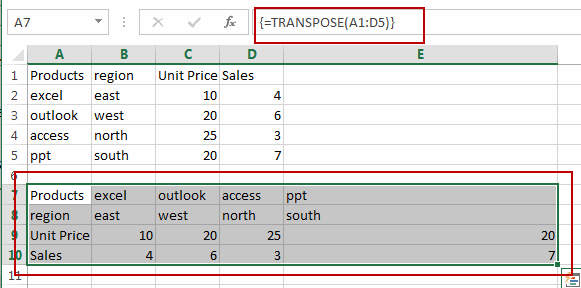## Convert Rows to Columns Using VBA

You can also use an Excel VBA Macro to achieve the same result of converting rows to columns. Just do the following steps:

#1 open your excel workbook and then click on “Visual Basic” command under DEVELOPER Tab, or just press “ALT+F11” shortcut.#2 then the “Visual Basic Editor” window will appear.

#3 click “Insert” ->”Module” to create a new module.#4 paste the below VBA code  into the code window. Then clicking “Save” button.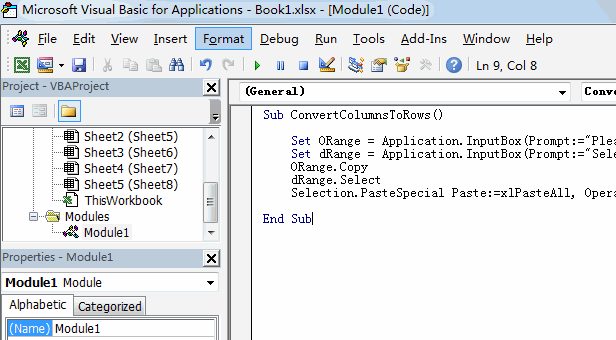```Sub ConvertColumnsToRows()
Set ORange = Application.InputBox(Prompt:="Please select one range that you want to transpose", Title:="ConvertColumnsToRows", Type:=8)
Set dRange = Application.InputBox(Prompt:="Select the first cell of the destination range", Title:="ConvertColumnsToRows", Type:=8)
ORange.Copy
dRange.Select
Selection.PasteSpecial Paste:=xlPasteAll, Operation:=xlNone, SkipBlanks:=False, Transpose:=True
End Sub```

#5 back to the current worksheet, then run the above excel macro. Click Run button.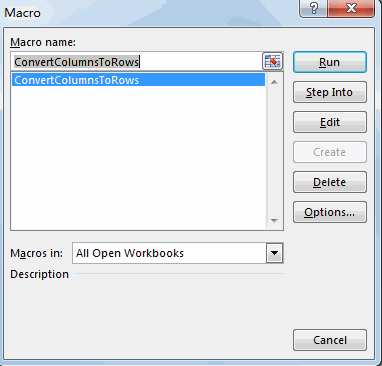#6 please select one range that you want to transpose. Click OK button.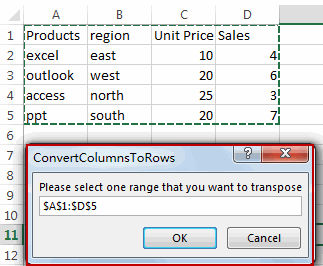#7 select the first cell of the destination range. Click OK button.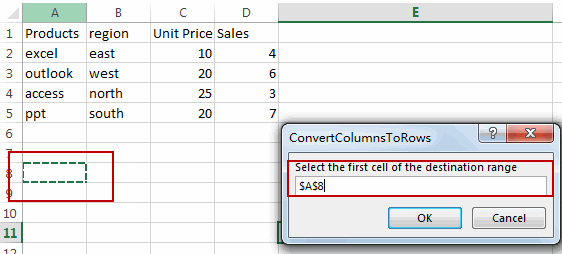#8 back to the current worksheet, then run the above excel macro. Click Run button.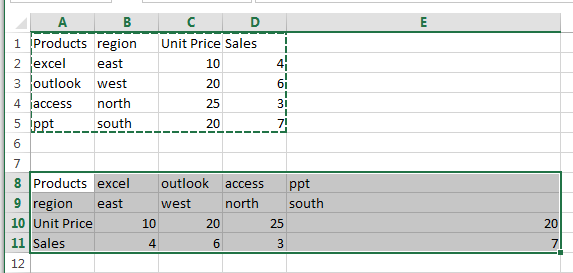### Related Functions

• Excel TRANSPOSE function
Excel TRANSPOSE formula allows you to rotate (swap) values from rows to columns and vice versa in Excel.The Excel TRANSPOSE Function syntax:=TRANSLATE (range) …

## How to Transpose Data from Columns to Rows

This post explains that how to transpose data from columns to rows, or from rows to columns in excel. if you want to rotate the data in columns of a worksheet to rearrange it in rows, you can use the “Paste Special Transpose” option to switch rows to columns or vice verse. you can also use the TRANSPOSE function to achieve the same result.

## Using Paste Special Transpose

To transpose the data in the range B2:B5 from columns to rows, refer to the following steps:

1# Select the range B2:B5 that you want to rearrange, then press CTRL+C to copy the selected Range.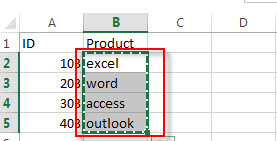2# Select Cell D2, then Right-click on the selected range, then click “Paste Special….”, the “Paste Special” window will appear.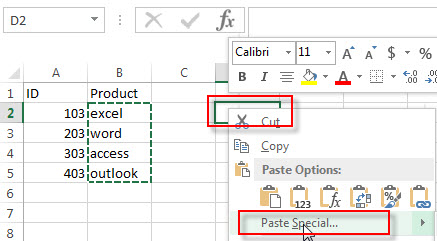3# checked “Transpose“. click OK.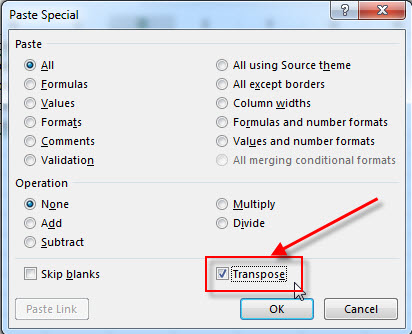4# let’s see the last result.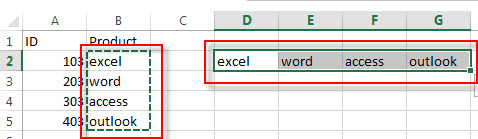## Using Transpose Function

If you want to transpose the range B2:B5 to D2:G2, just enter into the following array formula in Cell C1:

`=TRANSPOSE(B2:B5)`

You need to select the range D2:G2, then enter the above formula in the formula bar. Then click “Ctrl”+”Shift”+Enter key to convert its as an array formula.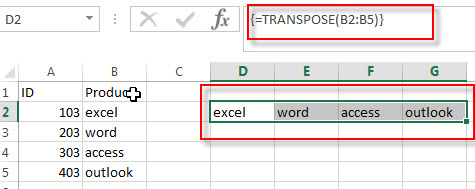### Related Functions

• Excel TRANSPOSE function
Excel TRANSPOSE formula allows you to rotate (swap) values from rows to columns and vice versa in Excel.The Excel TRANSPOSE Function syntax:=TRANSLATE (range) …